The ROBUSTREG Procedure
 Computational Resources

The algorithms for the various estimation methods need a different amount of memory for working space. Let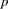be the number of parameters estimated andbe the number of observations used in the model estimation.

For M estimation, the minimum working space (in bytes) needed is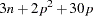If sufficient space is available, the input data set is also kept in memory; otherwise, the input data set is reread for computing the iteratively reweighted least squares estimates and the execution time of the procedure increases substantially. For each reweighted least squares,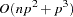multiplications and additions are required for computing the crossproduct matrix and its inverse. The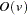notation means that, for large values of the argument,,is approximately a constant times.

Since the iteratively reweighted least squares algorithm converges very quickly (normally within fewer than 20 iterations), the computation of M estimates is fast.

LTS estimation is more expensive in computation. The minimum working space (in bytes) needed is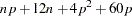The memory is mainly used to store the current data used by LTS for modeling. The LTS algorithm uses subsampling and spends much of its computing time on resampling and computing estimates for subsamples. Since it resamples if singularity is detected, it might take more time if the data set has serious singularities.

The MCD algorithm for leverage-point diagnostics is similar to the LTS algorithm.Previous Page | Next Page | Top of Page